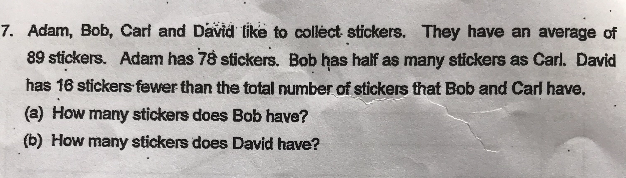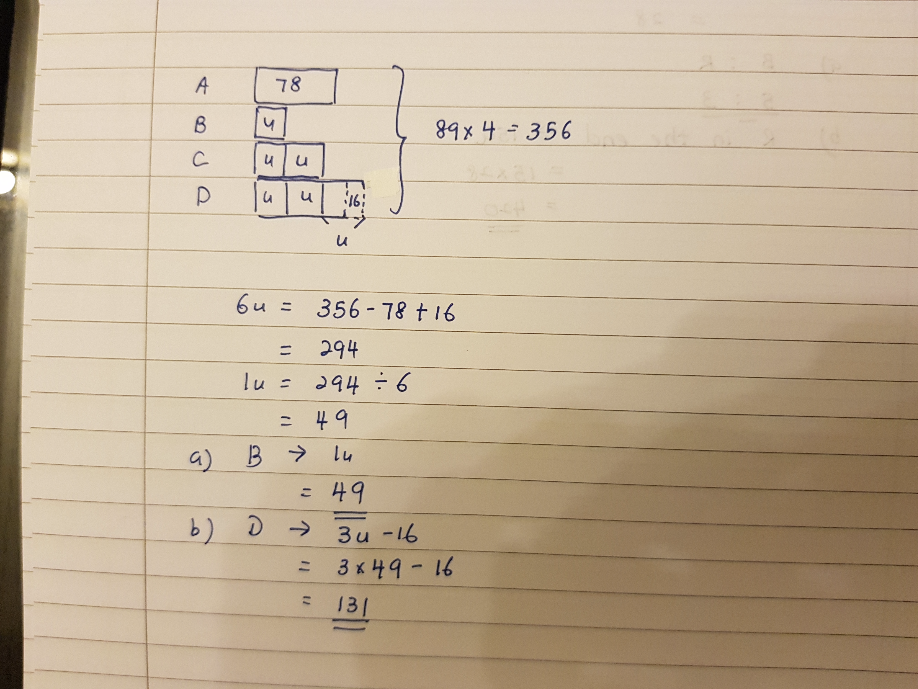# QuestionPls assist

Source: Tao Nan

Total number of stickers -> 89 × 4 = 356

A -> 78

B -> u

C -> 2u

D -> (B + C) – 16 = 3u – 16

A + B + C + D = 356

78 + u + 2u + 3u – 16 = 356

6u = 294

u = 49

(a) Bob has 49 stickers

(b) David has (3u – 16) = 131 stickers

0 Replies 1 Like ✔Accepted Answer0 Replies 1 Like